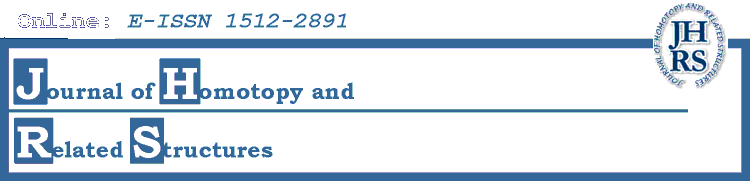# Volume 4 (2009), No. 1

1. Reparametrizations with given stop data

2. Nonisomorphic Verdier octahedra on the same base

3. Cobordism category of plumbed 3-manifolds and intersection product structures

4. On the classification of unstable $H^{\ast}V-A$-modules

5. Particle configurations and Coxeter operads

6. Rational self-homotopy equivalences and Whitehead exact sequence

7. OCHA and the swiss-cheese operad

8. The transfer in mod-p group cohomology between \Sigma_p \int \Sigma_{p^{n-1}}, \Sigma_{p^{n-1}} \int \Sigma_p and \Sigma_{p^n}

9. Reidemeister torsion and analytic torsion of spheres

10. Parallel Transport and Functors

11. On The Cofibrant Generation Of Model Categories

12. Ensembles simpliciaux règuliers